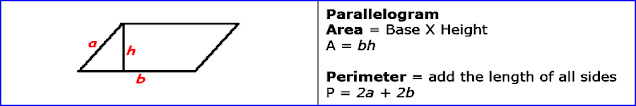# C++ Exercises

## C++ Program to calculate the area and perimeter of the parallelogram.

Let see the program to calculate the area and perimeter of the parallelogram.

```
Example:

Formula:parallelogram

```
```
#include <iostream>

using namespace std;
int main()
{
int base,height,width,area,perimeter;
cout<<"Enter base of parallelogram :";
cin>>base;
cout<<"Enter height of parallelogram :";
cin>>height;
cout<<"Enter width of parallelogram :";
cin>>width;
area= base*height;
perimeter= 2*(base+width);
cout<<"Area of parallelogram = :"<<area<<" sq. units."<<endl;
cout<<"Perimeter of parallelogram = :"<<perimeter<<" units."<<endl;
return 0;
}

```
##### Output:
```
Enter base of parallelogram :10
Enter height of parallelogram :4
Enter width of parallelogram :5

Area of parallelogram = :40 sq. units.

Perimeter of parallelogram = :30 units.

```